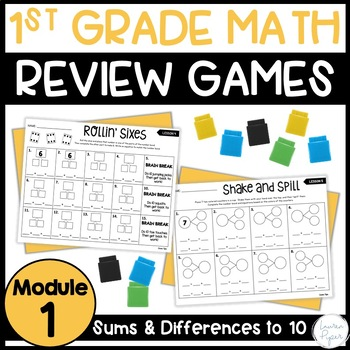# First Grade Eureka Math: Module 1 Games and Activities1st
Subjects
Standards
Resource Type
Formats Included
• PDF
Pages
194 pages

#### Also included in

1. This is the perfect supplemental product for your First Grade Eureka Math Curriculum! Included in this bundle are games and activities for EVERY SINGLE LESSON in the 1st Grade Eureka Curriculum. The activities can be used in whole group, small groups, or centers. They are easy prep and most activi
\$69.00
\$77.00
Save \$8.00
2. All you need for teaching First Grade Eureka Math Module 1 is in this BUNDLE! These supplemental resources for Eureka Math/Engage NY are student-friendly and align perfectly with the first grade curriculum. Included in this bundle: -First Grade Eureka Math Module 1 Games and Activities: A game for e
\$30.00
\$34.00
Save \$4.00

### Description

-It includes a game, activity, or craft for EVERY lesson in Grade 1 Module 1. That is 39 games!

-These activities are perfect to use for small group, whole group, or centers! They are easy prep and most games require little to no materials. Your kiddos will be engaged and excited to learn using these games!

-I use these for whole group instruction for almost every single lesson. My kids can't wait to see what game we are playing for math that day!

-Check out the preview to see examples of the activities and grab a free sample from lesson 8!

-You may purchase my other modules OR save big with the BUNDLE listed above!

Module 2

Module 3

Module 4

Module 5

Module 6

Do you need homework, review, or topic quizzes for your Eureka curriculum? Check out my First Grade Eureka Math Review and Assessments and be set for the whole year!

Total Pages
194 pages
N/A
Teaching Duration
N/A
Report this Resource to TpT
Reported resources will be reviewed by our team. Report this resource to let us know if this resource violates TpT’s content guidelines.

### Standards

to see state-specific standards (only available in the US).
Determine the unknown whole number in an addition or subtraction equation relating three whole numbers. For example, determine the unknown number that makes the equation true in each of the equations 8 + ? = 11, 5 = ▯ - 3, 6 + 6 = ▯.
Understand the meaning of the equal sign, and determine if equations involving addition and subtraction are true or false. For example, which of the following equations are true and which are false? 6 = 6, 7 = 8 - 1, 5 + 2 = 2 + 5, 4 + 1 = 5 + 2.
Add and subtract within 20, demonstrating fluency for addition and subtraction within 10. Use strategies such as counting on; making ten (e.g., 8 + 6 = 8 + 2 + 4 = 10 + 4 = 14); decomposing a number leading to a ten (e.g., 13 - 4 = 13 - 3 - 1 = 10 - 1 = 9); using the relationship between addition and subtraction (e.g., knowing that 8 + 4 = 12, one knows 12 - 8 = 4); and creating equivalent but easier or known sums (e.g., adding 6 + 7 by creating the known equivalent 6 + 6 + 1 = 12 + 1 = 13).
Relate counting to addition and subtraction (e.g., by counting on 2 to add 2).